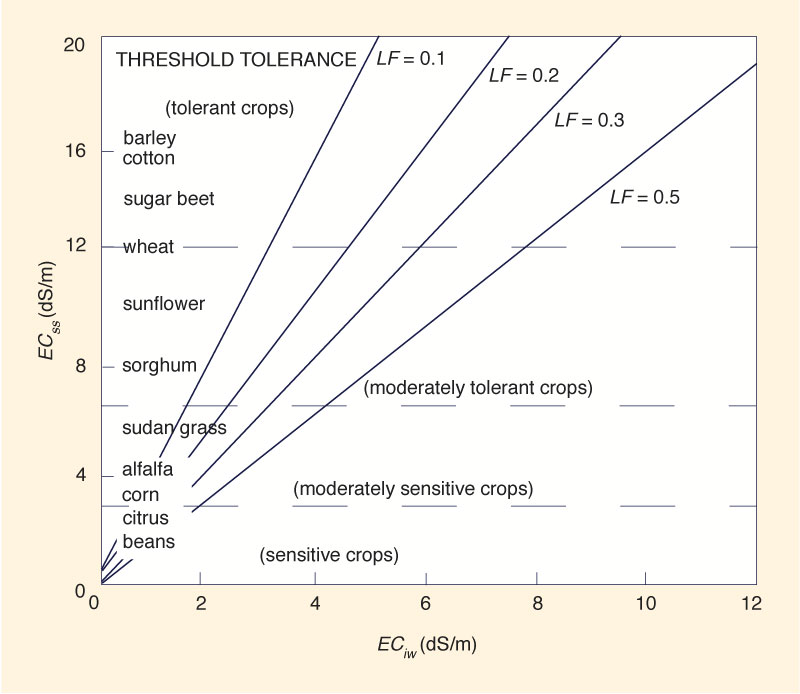ESTIMATION OF
THE LEACHING FRACTION
IN IRRIGATED AGRICULTURE

Source:

ASCE Manual 71, Second Edition, 2012.

1. DEFINITIONS OF LEACHING FRACTION

The leaching fraction is the ratio between the depth of drainage (water leaving the site) and the total depth of applied water (irrigation plus precipitation).

The leaching fraction is also defined as the ratio between the concentration of salts in the applied water (irrigation plus precipitation) and the concentration of salts in the drainage water. It must be noted that the irrigation water may be more saline than the precipitation water.

Also, the leaching fraction is the ratio of the electrical conductivity of the applied water to the electrical conductivity of the drainage water.

Figure 1 shows the leaching fraction LF required to avoid crop yield loss, given the electrical conductivity of the applied irrigation water ECiw (abscissas) and the electrical conductivity of the saturated soil extract ECss (ordinates).Fig. 1   Leaching fraction LF as a function of the electrical conductivity of the irrigation water
ECiw and the electrical conductivity of the saturated soil extract ECss.

For example, assume that tomatoes are to be grown in an arid region with irrigation water of electrical conductivity CEiw = 2 dS/m. Assume that the electrical conductivity of the soil is: ECss = 4 dS/m. Local precipitation is considered to be negligible.

From Fig. 1, with ECiw = 2 dS/m and ECss = 4 dS/m, the following leaching fraction is obtained: LF = 0.3. This means that 30% of the total applied water will have to be drained.

Example.

Let LF = leaching fraction, WL = amount of water to be leached, and CU = crop consumptive use of water. The amount WT of total applied water (irrigation plus precipitation plus amount of water to be leached) is:

WT = CU + WL

The leaching fraction is:

LF = WL / (CU + WL)

Thus:

WL = (LF × CU) / (1 - LF)

For instance, if the consumptive use of the crop is CU = 100 units and the required leaching fraction LF = 0.3, the amount of water to be leached is:

WL = (0.3 × 100) / (1 - 0.3) = 43

Thus, the amount of total applied water (to avoid yield loss) is:

WT = 100 + 43 = 143 units.

2. RELATIVE YIELD OF CROPS IN SALINE AREAS

Relative crop yield in saline areas (Yr) is expressed by the following relationship:

Yr = 1 - (b / 100) (CEss - a)

in which:

Yr = relative crop yield, in fraction of 1;

a = tolerance threshold to salts, a value applicable to a given crop, in dS/m;

b = slope of the line of yield loss, a value applicable to a given crop, expressed in percentage loss for each dS/m of electrical conductivity;

CEss = electrical conductivity of a saturated soil extract taken from the root zone (dS/m).

For example, for a crop with a = 4 dS/m and b = 20 (%), the relative yield for CE varying between 4.0 and 9.0 dS/m is the following:

CEss = 4 dS/m: Yr = 1.0

CEss = 5 dS/m: Yr = 0.8

CEss = 6 dS/m: Yr = 0.6

CEss = 7 dS/m: Yr = 0.4

CEss = 8 dS/m: Yr = 0.2

CEss = 9 dS/m: Yr = 0.0

Example.

Figure 2 shows a measured relative yield function applicable to corn, for which a = 3.6 dS/m, and b = 100/(10.8 - 3.6) = 100/7.2 = 13.9.

Thus, for CEss = 6.0 dS/m, the relative yield is: Yr = 1 - (13.9/100) (6.0 - 3.6) = 0.67.

For CEss = 7.5 dS/m, the relative yield is: Yr = 1 - (13.9/100) (7.5 - 3.6) = 0.46.Fig. 2   Relative yield of corn vs electrical conductivity of the soil.

 211116 09:00